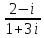# Honors Algebra 2 Journal Prompts 2

 Honors Algebra 2 Journal Prompts – 2nd Semester For each prompt assigned, write a well-developed, thorough essay-style response. Incorporate all relevant mathematical terminology and examples to illustrate your response. Your response should be crafted assuming your reader does not have the mathematical background of a teacher. Give an example of a quadratic function that has a maximum value. Explain how you know it has a maximum and how to find that maximum algebraically (not graphically). Find the maximum value for your function. Write an essay comparing the graphs of these functions: y = x2, y = (x+2)2 + 9, and y = -(x+2)2 + 9. Be sure to address these features in your response: direction of opening, vertex, equation of the axis of symmetry. If you are given the equation of the axis of symmetry of a parabola and the coordinates of the vertex, can you write the equation of the parabola? Explain why or why not. Explain how to find the equation for the quadratic function that has its vertex at (-1, 2) and contains the point (0, -1). Describe two ways to find the coordinates of the vertex of y = -2x2 + 8x – 15. Which of the following are complex numbers? Explain your answers. 5, 3i, -1 + 2i Explain how to simplify the quotient. Can you use synthetic division to divide a polynomial by x2 – 2? Why or why not? Explain how to use variable (u) substitution to find all of the roots of x4 – 34x2 + 225 = 0. When P(x) touches but does not cross the x-axis at (r, 0), what must be true of P(x). Given an example of a polynomial for which this is true. Is it possible for f(x) = 32x3 – 48x2 + 22x – 3 to have 0, 1, 2, 3, or 4 zeros between 0 & 1? Explain fully. Explain how, without solving a quadratic equation, you can determine if it has 1 real root or 2 real, rational roots, or 2 real, irrational roots, or 2 complex roots. Describe three different ways to solve x2 - 2x – 15 = 0. Which method do you prefer and why? Explain how the graph of the parent function y = x2 can be used to graph any quadratic function. Include a description of the effects produced by changing a, h, and k in the equation y = a(x – h)2 + k using values you choose for a, h, and k. Explain how you can determine the consistency and dependence of a system of equations without graphing the system. Describe three different ways to solve a system of two equations in two variables. Include an example system. Explain two ways to determine whether (-4, 6) is a solution to a system of inequalities. Explain how to use the correlation coefficient to determine how two quantities are related. Include examples. Describe the process of finding the equation of a line when you are given two points on the line. Incorporate examples. Explain how to solve x2 – 2x + 3 = 0 by completing the square. Download 8.81 Kb.Share with your friends: